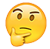So we listening to this Kacy Hill album or nah?

Started by YNW Squiddy, Jun 30, 2017, in Music

1. YNW Squiddy

where's the meat

Jun 30, 2017
I'm 30 seconds in and it's already better than Jay's tbh

1
Sqrt Sqrt likes this.
1
Sqrt Sqrt likes this.
2. YNW Squiddy

where's the meat

Jun 30, 2017
Keep Me Sane1
Sqrt Sqrt likes this.
1
Sqrt Sqrt likes this.
3. Jun 30, 20172
Sqrt Sqrt and YNW Squiddy like this.
2
Sqrt Sqrt and YNW Squiddy like this.
4. YNW Squiddy

where's the meat

Jun 30, 2017
Where's @Deadpool at I know he's listening to this

1
Sqrt Sqrt likes this.
1
Sqrt Sqrt likes this.
5. YNW Squiddy

where's the meat

Jun 30, 2017
You check this out yet?2
Sqrt Sqrt and Jehovah like this.
2
Sqrt Sqrt and Jehovah like this.
6. Jun 30, 2017

If this aint on Spotify its a wrap2
Sqrt Sqrt and YNW Squiddy like this.
2
Sqrt Sqrt and YNW Squiddy like this.
7. YNW Squiddy

where's the meat

Jun 30, 2017
I mean I'm pretty bummed that Travis isn't anywhere on this, but it sounds nice, imo

1
Sqrt Sqrt likes this.
1
Sqrt Sqrt likes this.
8. Jun 30, 2017
Found it Spotify clutch af when you can't be bothered downloading albums, so this is what streaming was made for2
Sqrt Sqrt and YNW Squiddy like this.
2
Sqrt Sqrt and YNW Squiddy like this.
9. Jun 30, 2017
This 2nd track pretty nice not gna lie2
Sqrt Sqrt and YNW Squiddy like this.
2
Sqrt Sqrt and YNW Squiddy like this.
10. YNW Squiddy

where's the meat

Jun 30, 2017
When the Latest Posts ticker updates before the New Reply Posted button shows up so you just sit there waiting for it like1
Sqrt Sqrt likes this.
1
Sqrt Sqrt likes this.
11. YNW Squiddy

where's the meat

Jun 30, 2017
Yea that beat might be the highlight of the project so you might as well turn it off after that2
Sqrt Sqrt and Jehovah like this.
2
Sqrt Sqrt and Jehovah like this.
12. Jun 30, 2017
welp im out2
Sqrt Sqrt and YNW Squiddy like this.
2
Sqrt Sqrt and YNW Squiddy like this.
13. YNW Squiddy

where's the meat

Jun 30, 2017
ok I lied, Clarity goes pretty hard

Tags: